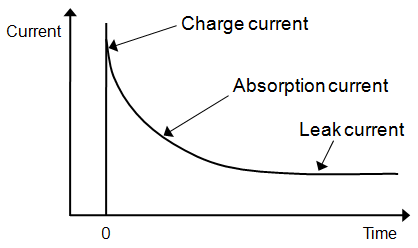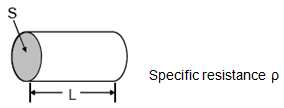Ceramic Capacitors FAQQWhat are the standards and units of insulation resistance values for chip multilayer ceramic capacitors?

A
The insulation resistance of a multilayer ceramic capacitor represents the ratio between the applied voltage and the leakage current after a set time (ex. 60 seconds) while applying DC voltage without ripple between the capacitor terminals. While the theoretical value of a capacitor's insulation resistance is infinite, since there is less current flow between insulated electrodes of an actual capacitor, the actual resistance value is finite. This resistance value is called "insulation resistance" and denoted with units such as Meg Ohms [MΩ] and Ohm Farads [ΩF].

Behavior of insulation resistance value

Directly after DC voltage is applied to a capacitor, the rush current, which is also called the charge current flows as shown in Figure 1. As the capacitor is gradually charged, the current decreases exponentially.Current I(t) flowing after time t passes are categorized into three types as seen in the equation (1) below, namely, charge current Ic(t),

absorption current Ia(t) and leakage current Ir. I(t)=Ic(t)+Ia(t)+Ir equation (1)

Charge current indicates current flowing through an ideal capacitor. Absorption current flows with a delay compared with the charge current, accompanying dielectric loss at a low frequency and the reverse polarization for high dielectric constant type capacitors (ferroelectric) and the Schottky barrier which occurs at the interface between the ceramics and the metal electrodes.

Leakage current is a constant current flowing after a certain period of time when the influence of absorption current diminishes.

Therefore, the value of the flowing current varies depending on the amount of time voltage is applied to the capacitor. This means that the capacitor's insulation resistance value cannot be determined unless the timing of the measurement after voltage application is specified.

Insulation resistance value

Insulation resistance value is represented in units Meg Ohms [MΩ] or Ohm Farads [ΩF]. Its specified value varies depending on the capacitance value. The value is specified as the product of nominal capacitance value and insulation resistance (CR product), for example over 10,000MΩ for 0.047µF and lower, and over 500ΩF for higher than 0.047µF.

Guaranteed insulation resistance value [example]

Specification Spec value Specification (1) Specification (2) Capacitance C≦0.047µF: over10000MΩ C＞0.047µF: over500ΩF 50ΩF or more Measured voltage: rated voltage Charge time: Two minutes Measured temperature: normal temperature Charge/discharge current: lower than or equal to 50mA Measured voltage: rated voltage Charge time: 1 minute Measured temperature: normal temperature Charge/discharge current: lower than or equal to 50mA

 Example of Equation In the case of 1µF Specification (1) Insulation Resistance "=500ΩF/1*10 -6 F" "=500Ω/1*10 -6 " "=500Ω*10 6 " " =500MΩ or more " Specification (2) Insulation Resistance "=50ΩF/1*10 -6 F" "=50Ω/1*10 -6 " "=50Ω*10 6 " " =50MΩ or more "

Representative
Capacitance
Specification (1)
Insulation Resistance
Specification (2)
Insulation Resistance
1μF 500MΩ or more 50MΩ or more
2.2μF 227MΩ or more 22.7MΩ or more
4.7μF 106MΩ or more 10.6MΩ or more
10μF 50MΩ or more 5MΩ or more
22μF - 2.27MΩ or more
47μF - 1.06MΩ or more
100μF - 0.5MΩ or more

As shown above, the higher capacitance value, the less its insulation resistance becomes. The reason is explained below. Insulation resistance can be figured out with the Ohm's law from applied voltage considering the multilayer ceramic capacitor as a conductor as well as electric current.

Resistance value R may be expressed with equation (2) with the length of the conductor as L, and the area of cross section as S and specific resistance as ρ.
R= ρ • L/S equation (2)Likewise, capacitance C can be represented with equation (3) by expressing distance between electrodes for the multilayer ceramic capacitor (dielectric thickness) as L, the area of inner electrode as S and the dielectric constant as ε.
C ∝ ε • S/L equation (3)
Equation (4) can be derived from equation (2) and equation (3) indicating that R and C are inversely proportional.
R ∝ ρ • ε/C equation (4)

Insulation resistance being higher indicates that the leakage current under DC voltage is lower. Generally, circuits are supposed to have higher performance when the insulation resistance value is higher.

Reference FAQ

> What are typical insulation resistance values for chip multilayer ceramic capacitors?

We appreciate your cooperation with the FAQ improvement questionnaire.